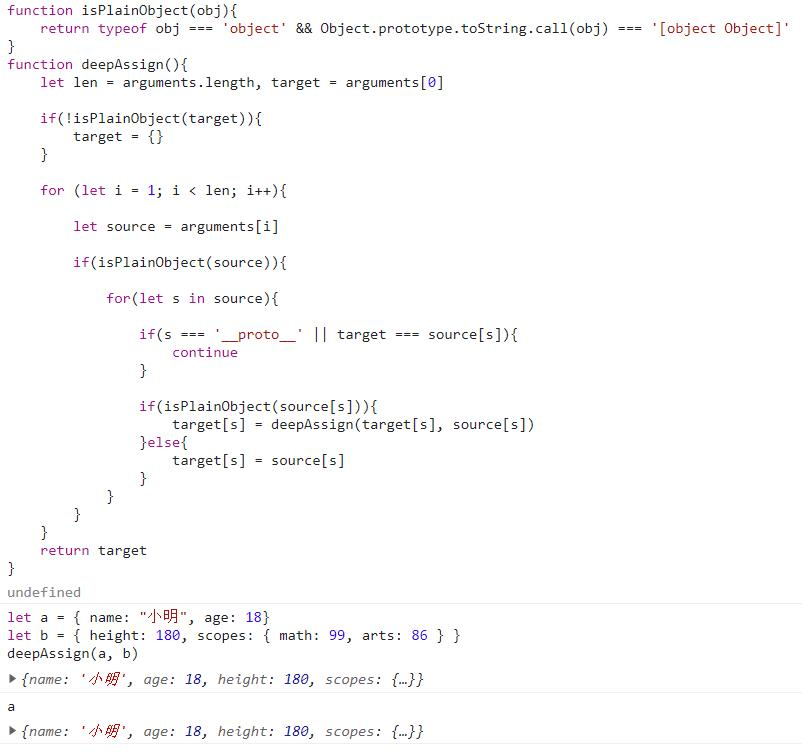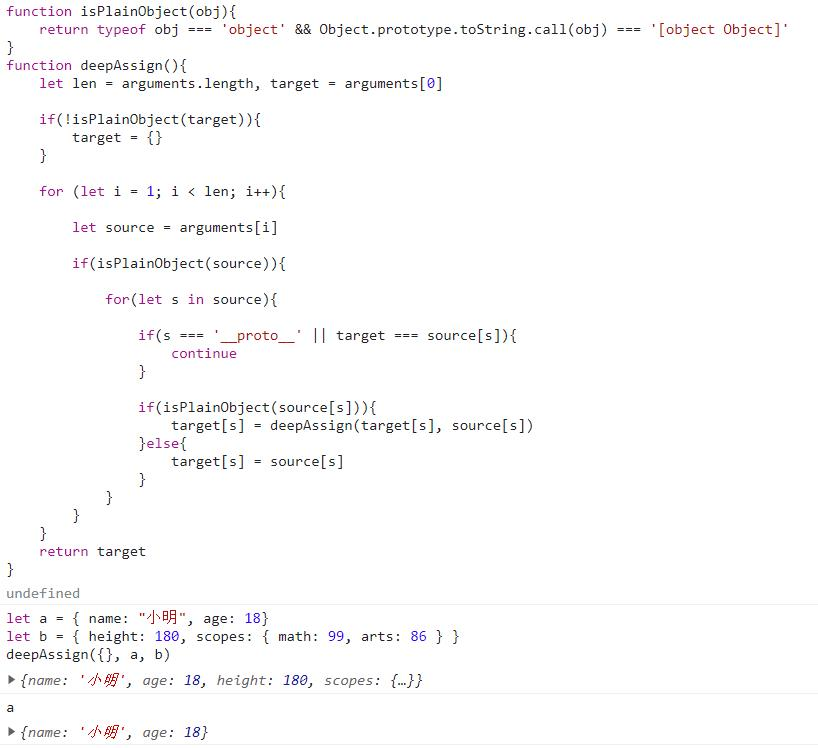# js对象深度合并（多参数），js合并对象的方法，json合并

js合并对象（json）有两种方法：
1、浅层合并；
2、深度合并；

## json对象的浅层合并方法

``````let newObj = Object.assign(obj1, obj2, obj3, ...)
``````

## 两个对象的深度合并方法

``````/**
*判断对象是否是一个纯粹的对象
*/
function isPlainObject(obj){
return typeof obj === 'object' && Object.prototype.toString.call(obj) === '[object Object]'
}
/**
*深度合并两个对象的方法
*/
function deepAssign(target, source){
if(isPlainObject(source)){
if(!isPlainObject(target)){
target = {}
}
for ( let s in source ) {
if ( s === '__proto__' || target === source[s] ) {
continue
}
if ( isPlainObject(source[s]) ) {
target[s] = deepAssign(target[s], source[s])
} else {
target[s] = source[s]
}
}
return target
}
}
``````

``````
/**
*判断对象是否是一个纯粹的对象
*/
function isPlainObject(obj){
return typeof obj === 'object' && Object.prototype.toString.call(obj) === '[object Object]'
}

/**
*深度合并多个对象的方法
*/
function deepAssign( ){
let len = arguments.length, target = arguments
if(!isPlainObject(target)){
target = {}
}
for (let i = 1; i < len; i++){
let source = arguments[i]
if( isPlainObject( source ) ){
for( let s in source ){
if ( s === '__proto__' || target === source[s] ) {
continue
}
if( isPlainObject( source[s] ) ){
target[s] = deepAssign(target[s], source[s])
}else{
target[s] = source[s]
}
}
}
}
return target
}
``````

• 用法
``````let newObj = deepAssign(obj1, obj2, obj3, ...)
``````

## 实现多参数对象深度合并的优势

``````let a = { name: "小明", age: 18}
let b = { height: 180, scopes: { math: 99, arts: 86 } }
deepAssign(a, b)
````````````let a = { name: "小明", age: 18}
let b = { height: 180, scopes: { math: 99, arts: 86 } }
deepAssign({}, a, b)
``````734802480（已满）、 794324979

﻿